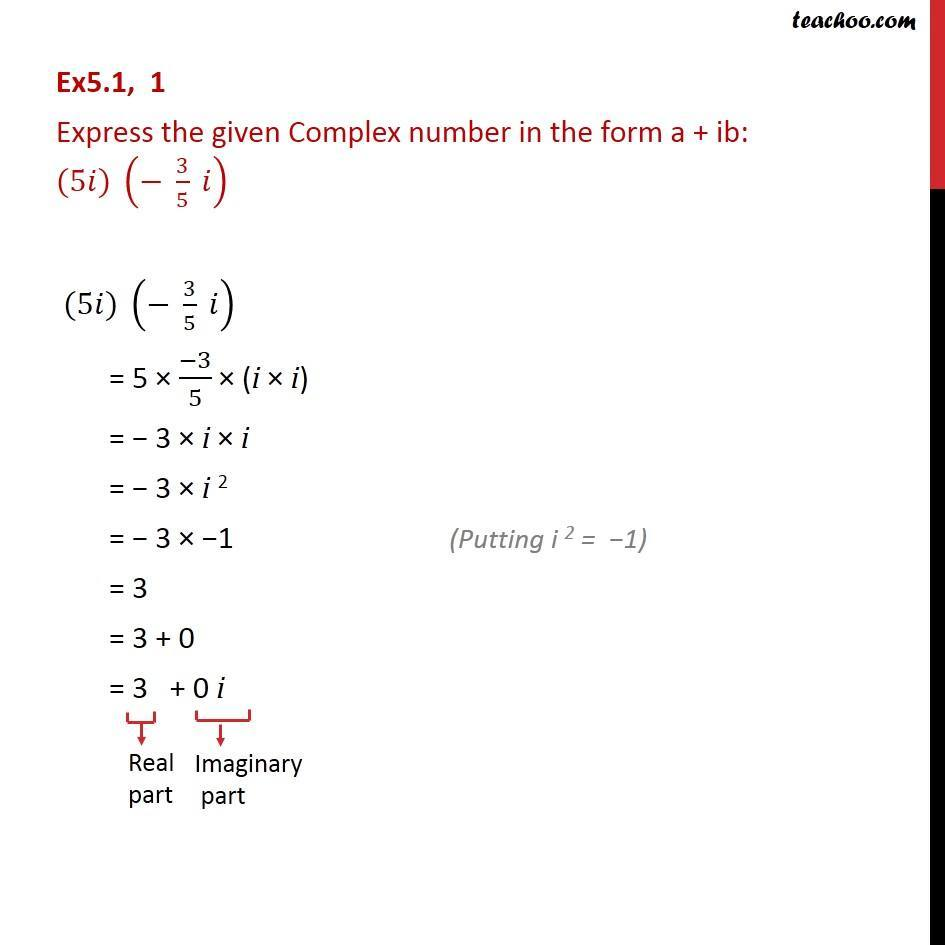1. Chapter 5 Class 11 Complex Numbers (Term 1)
2. Serial order wise
3. Ex 5.1

Transcript

Ex5.1, 1 Express the given Complex number in the form a + ib: (5 ) ( 3/5 ) (5 ) ( 3/5 ) = 5 ( 3)/5 (i i) = 3 i i = 3 i 2 = 3 1 = 3 = 3 + 0 = 3 + 0 i